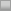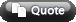View Single Post01-20-2008, 04:07 PM #3 The Bryce Stoxpoker Sponsored Forum   Join Date: Oct 2005 Location: Edmonton Posts: 4,245 Re: Maximizing EV to Find a GTO Betsize Ah, very good. I'm kind of doing the tour de force of mucking everything up in trying to get familiar with the higher end math stuff, but that's good. Let me just talk through the unsimplified equation first so that I can make sure I'm understanding it correctly: EV = n*-s The amount we lose when our opponent calls us with the nuts... + (1/(s+1) - n)*s ... plus the amount we win a bet when our opponent calls us without the nuts equals our EV. I was a little stuck in trying to figure out why were weren't taking into account how often we won the pot by bluffing, but then I realized that if we're indifferent to bluffing the EV on our bluffs is 0. Am I correct in my line of thinking there? So if were going to add equity into this what that does is change our point of indifference when bluffing. Say we are betting 1 unit into a pot of 2 units, in a nuts or bluff scenario our point of indifference when bluffing is if our opponent calls 33% of the time. If we only lose the pot 85% of the time when bluffing (15% equity), however, our point of indifference becomes 0.33/.85=0.388. So we could represent this in the equation: 1/(s+1)/o Where o is the % our opponent wins when we're bluffing. Now, I know that this is a pretty clunky solution, since if our opponent's range is split into something like the nuts and bluff catchers the amount we're bluffing affects our average equity with our bluffs when called and vice versa, but for the sake of functionality it actually would be fairly reasonable, since you would only have to tweak the numbers back and forth a few times until you were fairly close to on the money. What we could further do, if the above is true, is change n in the equity equation so that it represents our opponent's average equity when calling us. Am I on track so far?